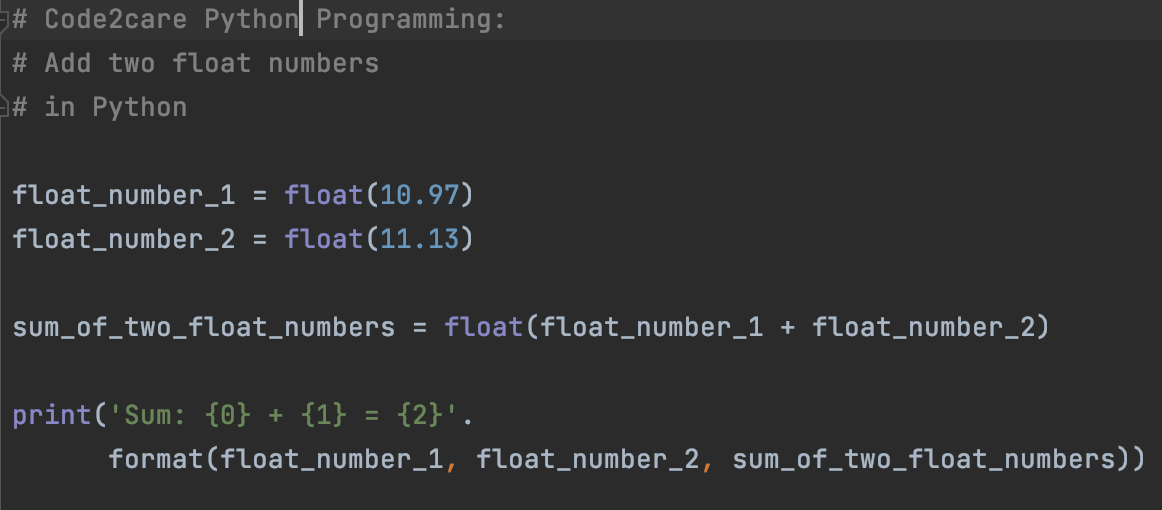# How to add two float numbers in Python

Let's see how we can add two float numbers in Python using a program.

``````# Code2care Python Programming:
# in Python

float_number_1 = float(10.97)
float_number_2 = float(11.13)

sum_of_two_float_numbers = float(float_number_1 + float_number_2)

print('Sum: {0} + {1} = {2}'.format(float_number_1, float_number_2, sum_of_two_float_numbers))``````

PyCharm Output:
/Users/code2care/.local/share/virtualenvs/examples-E9HN9Crc/bin/Python /Users/code2care/PycharmProjects/examples/main.py
Sum: 10.97 + 11.13 = 22.1

Process finished with exit code 0